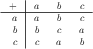# Latex multicolumn is not recognized

HI

R0 = GF(3)
print R0.multiplication_table()

edit retag close merge delete

Sorry John Palmieri, Yes, I forgot to precise :OS Ubuntu 18.04, notebook with SageMath 8.9

Sort by » oldest newest most voted

Well...

sage: latex(GF(3).multiplication_table())
{\setlength{\arraycolsep}{2ex}
\begin{array}{r|*{3}{r}}
\multicolumn{1}{c|}{\ast}&a&b&c\\\hline
{}a&a&a&a\\
{}b&a&b&c\\
{}c&a&c&b\\
\end{array}}


In a console (or in emacs's sage-shell-view), view(GF(3).addition_table()) gets me this:Sage's view uses Mathjax by default, but reverts to $\LaTeX$ when the latex string contains some "trigger" commands (this can be customized via latex.add_to_mathjax_avoid_list).

But, indeed, in a Jupyter notebook, this fails (not only on \multicolumn but also on {\setlength{\arraycolsep}{2ex}), probably because the notebook can't use $\LaTeX$\, and mathjax isn't a complete implementation if it).

By the way, the mathjax implementation of the present server has the same problem: A cut'n'paste of the $\LaTeX$ string generating the (correct) representation of the multiplication table gives this:

$${\setlength{\arraycolsep}{2ex} \begin{array}{r|*{3}{r}} \multicolumn{1}{c|}{\ast}&a&b&c\\hline {}a&a&a&a\ {}b&a&b&c\ {}c&a&c&b\ \end{array}}$$

This can probably worked around by saving the latex text, compiling it with an external LaTeX installation, converting the output to, say, .png and displaying it in the notebook. An utility function might be written for that.

One more reason to use $\LaTeX$ an Sagetex (or pythontex (or org-mode))...

HTH,

more

What is the point of \multicolumn{1}{...}, or at least what is its point here? Can we change this to make it compatible with MathJax?

It affects alignment for that one cell, but I think we can have slight misalignments (which may only be relevant if the terms in the table have names with more than one character) to gain better functionality in the Jupyter notebook.

LaTeX rendering in the Jupyter notebook is performed by MathJax. Unfortunately, MathJax does not support multicolumn, see https://docs.mathjax.org/en/v2.7-late... , in particular the sentence MathJax only implements a limited subset of the array environment’s preamble, i.e., only the l, r, c, and | characters alongside : for dashed lines; everything else is ignored.

more

Nils, your answer is perfectly valid and correct. Just less verbose than mine...